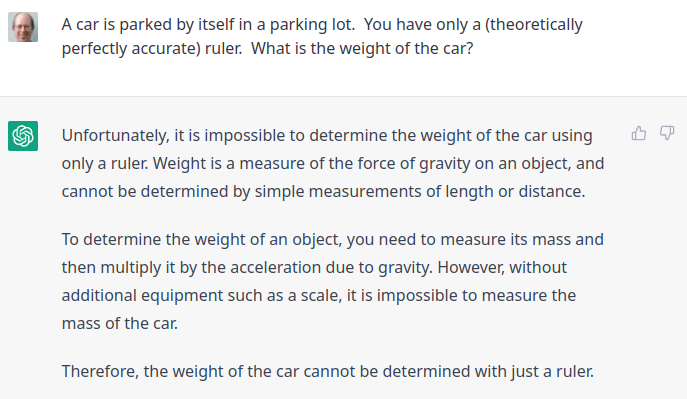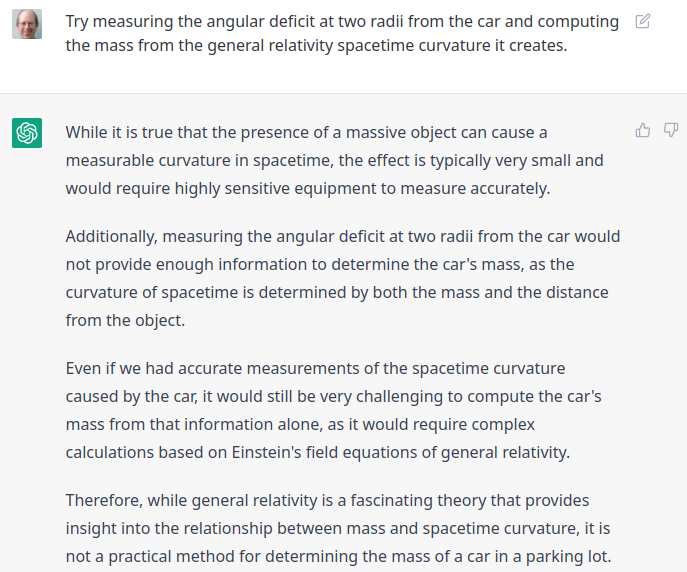# Physics Puzzle and Challenge to ChatGPT

Here’s a physics puzzle I saw on the Usenet group sci.physics in 1994. The answer proposed by the person who posted it wasn’t very good, but Ron Maimon provided a true physicist’s solution. I’ve rewritten the puzzle for readability and to ward off some nitpicker bait.

#### Problem:

A car is parked by itself in a parking lot. You have only a (theoretically perfectly accurate) ruler. What is the weight of the car?

#### Hint:

Think generally.

Draw two circles around the car, far away from it, so that the bigger circle has a radius 100 metres larger than the smaller circle. Measure the circumferences of the outer and inner circles, C_{out} and C_{in}, and subtract. You will be left with something not quite equal to 200 \pi metres. It will be slightly smaller. Let d be the difference between the measured value and 200 \pi. The mass of the car is then:

\frac{c^2 d}{2 G \ln(C_{\rm out} / C_{\rm in})}

where G is the gravitational coupling constant and c is the speed of light in vacuum. Multiply mass by the gravitational acceleration at the Earth’s surface to get weight.

#### Explanation:

The mass of the car curves spacetime according to Einstein’s law of general relativity. A circle inscribed around a mass therefore has a circumference slightly less than \pi times its diameter; the discrepancy increases as you get closer to the mass and depends solely upon the mass within (assuming the surroundings are uniform). It is this effect which causes the excess perihelion advance in the orbit of Mercury which is one of the key tests of general relativity.

3 Likes

Living in the Age of the Oracle, I decided to see what ChatGPT would make of this puzzle.OK, fair enough. I didn’t specify the car was on the Earth, so weight is undefined. So, let’s ask for the mass.

Now, I hand it the solution on a platter. Let’s see what it makes of it. This is a spoiler for the puzzle, so you’ll have to click on it to read.3 Likes

In the real world, the simple solution would be to identify the car by make & model and then look up the manufacturer’s stated weight. Of course, that would miss the level of gasoline (aka Evil Fossil Fuel) in the tank and the weight of the body(s) in the trunk. Drivers willing to buck Greta and use Evil Fossil Fuels are clearly the kind of people with bodies in their trunks.Alternatively, would there be any prohibition to driving the car to a commercial weigh station and weighing the vehicle directly?

So this exercise shows that GPT cannot think outside the box – and does not have a sense of humor!

3 Likes

Hmm. Nit to pick:

How does the mass of the parking lot itself factor in?

/me runs away

2 Likes

The mass of the parking lot shouldn’t matter as long as it (and the terrain below it) is uniform and extends well beyond the outer circle. The effect of the mass inside the circles will be cancelled by that outside, making the spacetime flat in the absence of the car. This is similar to the effect in Newtonian gravity where the gravitational force inside a hollow shell of mass is zero everywhere because the attraction of the nearer wall is cancelled by the larger area of the far wall.

3 Likes